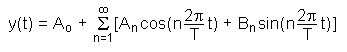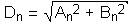The SDL Component Suite is an industry leading collection of components supporting scientific and engineering computing. Please visit the SDL Web site for more information....## Magnitude

 Unit: SDL_fourier Class: TFastFourier Declaration: property Magnitude[ix: integer]: double;

The readonly array property Magnitude provides access to the magnitude spectrum. The index ix may assume values between 0 and SpectrumSize div 2. Accessing any value of the array below 0 and above SpectrumSize div 2 will result in a zero value on read. The constant coefficient a0 is stored in Magnitude, the first harmonic in Magnitude, the second in Magnitude, and so on.

 Hint: The Magnitude is the square root of the sum of the squared coefficients. Assuming that the Fourier series is determined by the following equation,then the magnitude coefficients Dn are calculated as follows:Example: This property is used in the following example programs (see http://www.lohninger.com/examples.html for downloading the code): fft60hz, fft, ftfilter

Last Update: 2012-Oct-20